×

Search anything:

# Implementation of BERT

#### Machine Learning (ML) BERTGet this book -> Problems on Array: For Interviews and Competitive Programming

In this article, I tried to implement and explain the BERT (Bidirectional Encoder Representations from Transformers) Model .This article mainly consists of defining each component's architecture and implementing a Python code for it.

## BERT Model Architecture:

I have discussed in detail about the BERT model architecture in this article but in short , you can understand it as a number of encoder layers stacks on each other taken from Transformers architecture.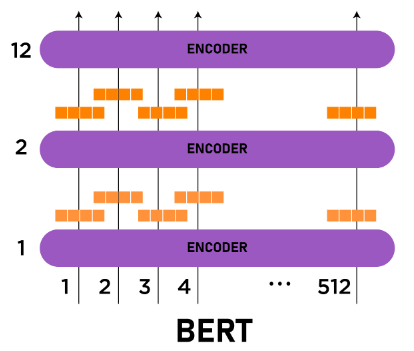## Model Building

For building a BERT model basically first , we need to build an encoder ,then we simply going to stack them up in general BERT base model there are 12 layers in BERT large there are 24 layers .So architecture of BERT is taken from the Transformer architecture .Generally a Transformers have a number of encoder then a number of decoder but BERT only uses the encoder part of the Transformer in its architecture . It is a little ambiguous why an encoder model performs well compared to an encoder-decoder language modeling .So let's see the architecture of an encoder and then try to implement it.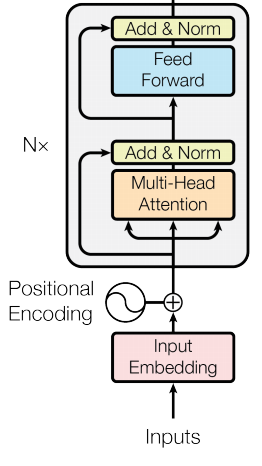In the above figure the input embeddings which we obtained after tokenizing and converting it into proper vector form which represent the words within the sentences or sequences with some numerical value feed into the Multi-headed attention layer after concatenating with positional embedding then the softmax output of the attention layer feed into the Feed forward neural networks which do its job of finding contextual information so the data flow somewhat should look like as depicted in the above diagram the decoder portion have nothing to do with BERT model so we are not going to discuss it.

So if we want to divide the architecture of Transformer into lower level we can say there are mainly three main components apart from input embedding:

• Feed Forward Neural Network
• Positional embedding

First we need to understand self-attention , so basically self attention takes account of all the other words in defining the meaning of a particular word. Lets see how to calculates it.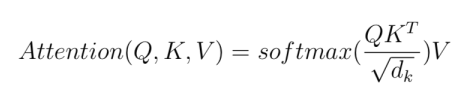#### Calculating self-attention:

We have to create three vectors query , key and value from the input embedding ,then we can calculate self attention by following the formula.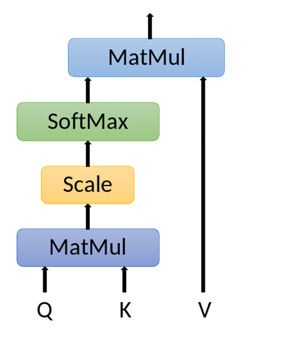Most of code provided here are component's code and are somewhat gives you a rough sense at high level , you can get full code here

``````"""
Baisc code for calculating attention or self-attention by dot product.
"""
def Attention(query, key, value):

scores = torch.matmul(query, key.transpose(-2, -1)) \
/ math.sqrt(query.size(-1))

scores = F.softmax(scores, dim=-1)
``````

So now you will able to understand Multi-headed attention .When Self-attention is calculated multiple times in the BERT architecture , It is therefore known as Multi-head Attention.The main behind this to calculate different contextual information in parallel in seperate attention layers , so it doesn't over saturate the single attention layer.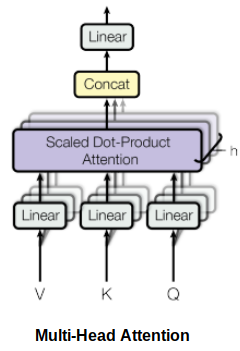``````
let's see it in code how it's look like. You can get full code [here]
"""
Model size and Number of heads(heads represent here number of inner layer).
"""

def __init__(self, h, dim_m):
super().__init__()

# d_v is the value vector or layer dimension
# d_k is the key vector or layer dimension
# d_k=d_v always

self.d_k = dim_m // h
self.h = h

self.linear_layers = nn.ModuleList([nn.Linear(dim_m, dim_m) for _ in range(3)])
self.output_linear = nn.Linear(dim_m, dim_m)
self.attention = Attention()

def forward(self, query, key, value):
batch_size = query.size(0)

# 1) Doing almost all the linear projections required in batch from d_model: (h x d_k)
query, key, value = [l(x).view(batch_size, -1, self.h, self.d_k).transpose(1, 2)
for l, x in zip(self.linear_layers, (query, key, value))]

# 2) Applying attention on all vecotors received after positional encoding.
x, attn = self.attention(query, key, value)

# 3) Concatnating the final layer + dense for getting the final output in same dimension as input.
x = x.transpose(1, 2)

return self.output_linear(x)
``````

#### Feed Forward neural network:

Feed Forward neural network helps a lot in finding the more contextual information related to particular pairs of words in sequences . It is not very clear why we need another feed forward network if we already have an attention layer which does the same job but it helps to improve the accuracy of the model you can see here if you wanna know more detail about it.

#### Positional encoding:

It contains the positional information about the sequence as well as the relative positional information regarding pairs of words. it helps the model not to get lost about relative positions.

``````class Encoder_block(nn.Module):
"""
Encoder block here represent the Encoder block here .
Encoder block here equivalent to the summation of MultiHead_Attention     ,Feed_Forward and other layers .
"""

"""
Following Pseudocode represnt simply the data flow in the encoder block.
"""

super().__init__()
self.feed_forward = feed_forward()
self.input_layer = layer2_Connection()
self.output_layer = layer2_Connection()

def forward(self, x):
x = self.input_layer()
x = self.output_sublayer(x, self.feed_forward)
return x
``````

## Training:

BERT is a language model which is trained in unsupervised fashion with a huge corpus in multiple languages . The reason for unsupervised or semi-supervised is that most of the language models trained in supervised learning (generally they are trained in such a way that they have to predict the next words in sequence which is their prediction goal ) , due to their supervised learning approach they lack contextual learning ability . BERT training consists of these two following two approaches .

Before training the BERT model , we simply replace about 15% of the words from the corpus with [Mask] token and during training we try to predict the value of the [Mask] for this to predict the model able to learn about the contextual or semantic information about the corpus.As it can used the data information from both sides left and right of the [Mask] token it explain the BERT model bidirectional property.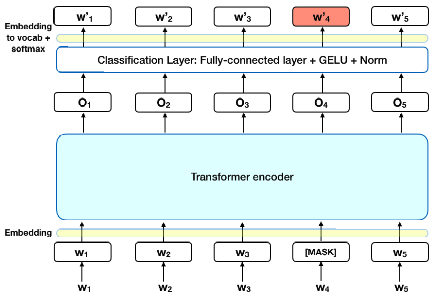As the above diagram shows we simply replaced the final layer with classification and trained it with a classification task.

``````	class Masked_Language_Model(nn.Module):
"""
Predicting the word in the original token by BERT model
"""

def __init__(self, hidden, vocab_size):
"""
parameter hidden refer to output size of BERT model
parameter vocabsize refers total vocab size of the corpus.
"""
super().__init__()
# passing through the BERT model
self.linear = nn.Linear(hidden, vocabsize)
# adding softmax layer to classify the token value
self.softmax = nn.LogSoftmax(-1)

def forward(self, x):
return self.softmax(self.linear(x))
``````

### 2.Next Sentence Prediction (NSP)

Another approach used to train the model is by simply shuffling a portion of a bunch of sentences within a corpus then trying to predict whether a given sentence follows the provided first sentence . Basically we are trying to predict whether a sentence is the next sentence or not.

We simply feed two sentences separated by [SEP] token and replace last layer with single classification bit which predict whether the following sentence is next or not.

``````class Next_Sentence_Prediction(nn.Module):
"""
classifying whether sentence is next or not.
"""

def __init__(self, hidden):
"""
parameter hidden represent BERT model size it may be 12 or 24.
"""
super().__init__()
# passing through BERT model
self.linear = nn.Linear(hidden, 2)
# concating a classification layer
self.softmax = nn.LogSoftmax(-1)

def forward(self, x):
return self.softmax( self.linear(x[:, 0]) )
``````

After training on these two tasks the word embedding obtained from that are more contextually informative or rich as compared to others word embedding obtained from different models , the model also able to orient itself into such a position that it is able to find more deep semantic or contextual meaning within text due to its architecture . We are not able to cover the whole training part as it requires in- depth knowledge of custom tokenizers , working on GPUs or TPUs , etc.

## Question

#### Although the concept of Self-attention and Multi-headed attention are same but why we need to use multi-headed attention instead of self attention?

Multi headed attention provide model ability to learn number of different context at the same time.
Because the computation Power required for self attention is more than multi-headed attention.
Because multi headed attention runs parallelly.
Above statement is not true self attention used more.
As multi-headed attention allows the model to learn different context between different pairs of words.

With this article at OpenGenus, you must have a good understanding of implementing BERT. Enjoy.#### Aryanshu Verma

Student at Indian Institute of Information Technology (IIIT), Gwalior | Intern at OpenGenus

Implementation of BERT